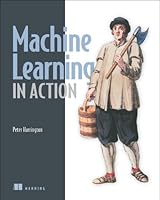# Machine Learning in Action## Book Description

Summary

in Action is unique book that blends the foundational theories of machine learning with the practical realities of building tools for everyday data . You'll use the flexible Python programming language to build programs that implement algorithms for data classification, forecasting, recommendations, and higher-level features like summarization and simplification.

A machine is said to learn when its performance improves with experience. Learning requires algorithms and programs that capture data and ferret out the interesting or useful patterns. Once the specialized domain of analysts and mathematicians, machine learning is becoming a skill needed by many.

Machine Learning in Action

is a clearly written tutorial for developers. It avoids academic language and takes you straight to the techniques you'll use in your day-to-day work. Many (Python) examples present the core algorithms of statistical data processing, data analysis, and data in you can reuse. You'll understand the concepts and how they fit in with tactical tasks like classification, forecasting, recommendations, and higher-level features like summarization and simplification.

Readers need no prior experience with machine learning or statistical processing. Familiarity with Python is helpful.

What's Inside

• A no-nonsense introduction
• Examples showing common ML tasks
• Everyday data analysis
• Implementing classic algorithms like Apriori and Adaboos

PART 1 CLASSIFICATION
Chapter 1. Machine learning basics
Chapter 2. Classifying with k-Nearest Neighbors
Chapter 3. Splitting datasets one feature at a time: decision trees
Chapter 4. Classifying with probability theory: naïve Bayes
Chapter 5. Logistic regression
Chapter 6. Support vector machines
Chapter 7. Improving classification with the AdaBoost meta

PART 2 FORECASTING NUMERIC WITH REGRESSION
Chapter 8. Predicting numeric values: regression
Chapter 9. Tree-based regression

PART 3 UNSUPERVISED LEARNING
Chapter 10. Grouping unlabeled items using k-means clustering
Chapter 11. Association analysis with the Apriori algorithm
Chapter 12. Efficiently finding frequent itemsets with FP-growth

Chapter 13. Using principal component analysis to simplify data
Chapter 14. Simplifying data with the singular value decomposition
Chapter 15. Big data and MapReduce

## Book Details

• Title: Machine Learning in Action
• Author:
• Length: 384 pages
• Edition: 1
• Language: English
• Publisher:
• Publication Date: 2012-04-28
• ISBN-10: 1617290181
• ISBN-13: 9781617290183